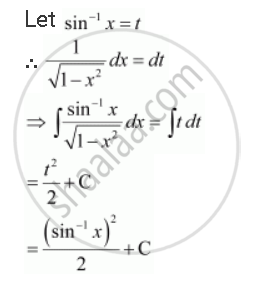Share

# Integrate the Functions (Sin^(-1) X)/(Sqrt(1-x^2)) - CBSE (Commerce) Class 12 - Mathematics

ConceptMethods of Integration - Integration by Substitution

#### Question

Integrate the functions (sin^(-1) x)/(sqrt(1-x^2))

#### SolutionIs there an error in this question or solution?

#### Video TutorialsVIEW ALL 

Solution Integrate the Functions (Sin^(-1) X)/(Sqrt(1-x^2)) Concept: Methods of Integration - Integration by Substitution.
S